# Logarithms

## Overview

The logarithm of a number x is the exponent (i.e. power) y by which another number (known as the base, b) must be raised in order to produce x. The base used can vary, depending on the area of application. In computer science, a base of two is often used, while in pure mathematics and many scientific applications a base known as e is used (more about this later).

In engineering and electronics, base ten is frequently used, and is obviously well suited for use with a decimal number system. For example, the logarithm to base ten of one thousand will be three, because one thousand is ten raised to the third power. The relationship can be clearly seen by studying the following expressions:

103 = 1,000

log10 1,000 = 3

This relationship can be expressed algebraically as follows:

b x = y

logb y = x

In the above expressions, the value of base b is fixed according to the application area as we have already mentioned, although it is possible to convert logarithmic expressions from one base to another. In the first expression, x is the exponent (or index or power) that determines how many instances of b will be multiplied together to obtain the result y.

In the second expression, x is the logarithm to base b of y. Clearly then, a logarithm is simply an exponent, as we have already said. For a given base, however, logarithms allow us to express very large values in a very concise way. To illustrate this point, here are some values to base ten represented as logarithms:

Values to Base 10 as Logarithms
ValueAs 10 raised to a powerLog to base 10
110 00
1010 11
10010 22
1,00010 33
10,00010 44
100,00010 55
1,000,00010 66
10,000,00010 77
100,000,00010 88
1,000,000,00010 99

As we can see from the above table, an increase in the logarithmic value (for base ten) of just one represents a tenfold increase in the value represented. This can be a very useful characteristic when we have to deal with values that can extend over a very large range, as we shall see. What should also be apparent from the table however, is that for a given range of numbers (for example, the range one to ten) a potentially infinite number of logarithmic values would be required to represent every possible real number value in that range.

In reality, the number of logarithmic values published in so-called log tables is limited due to practical constraints. This limitation will determine the precision with which logarithms can be used to perform calculations. A page from a book of logarithmic tables authored by George William Jones and published in the US circa 1889 is reproduced below. The entire publication (which is some eighty or so pages in total) can be downloaded in PDF format from the California Digital Library at: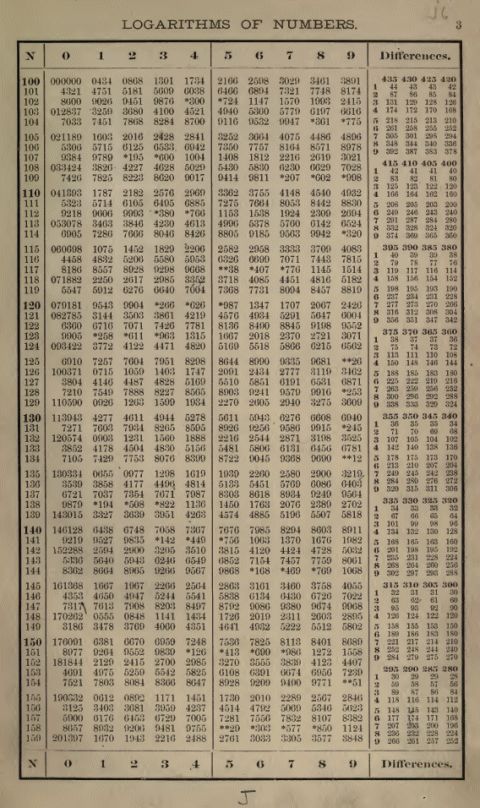A page from a book of logarithmic tables circa 1889

The underlying principles of logarithms were formulated hundreds of years ago as the result of the studies of a number of mathematicians over a long period of time. Much of the credit for bringing these principles to the attention of a wider audience, however, must go to a Scottish mathematician called John Napier.

Napier published his work on logarithms in 1614 and was thus instrumental in bringing about their widespread use for simplifying mathematical calculations. Logarithmic values were published in books containing comprehensive tables, and a simple mechanical device called a slide rule could be used to perform calculations using the properties of logarithms.

The slide rule was based on various sliding scale devices developed in the early 17th century, and its invention in 1622 is credited to English mathematician William Oughtred. Indeed the slide rule continued to be used by engineers and scientists to perform calculations right up until the 1970s, when it was finally rendered obsolete by the invention of the electronic calculator.

As with log tables (which were also rendered obsolete by the calculator), the accuracy of the results obtained with the slide rule was constrained by the fact that relatively large number ranges were represented using a relatively small number of divisions marked on the sliding scales. Add to this limitation the skill and accuracy with which the scales could be positioned and read by the operator. Indeed, the trade off between using log tables or a slide rule and carrying out a somewhat more rigorous process of calculation was that some degree of accuracy had to be sacrificed in return for a much faster method of calculation.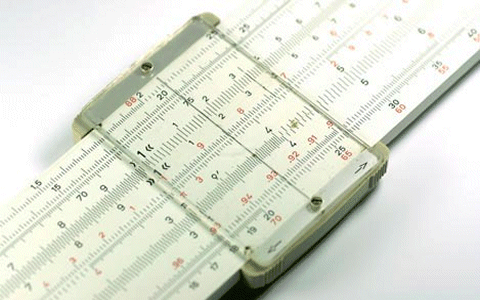The slide rule could speed up complex calculations

Today, we have calculators and computers that can perform complex calculations quickly and accurately. Logarithms are still useful, however, and a working knowledge of how they are used is essential for work or study in many fields, including nearly all branches of science and engineering.

You will already have come across values expressed in logarithmic terms many times, even if you are not conscious of the fact. One all too familiar example is the Richter Scale, which is a base-10 logarithmic scale used to measure the strength of earthquakes (or to be precise, the amplitude of the seismic waves that they produce).

The amplitude of the waves produced by an earthquake that measures 5.0 on the Richter Scale is ten times the amplitude of those produced by an earthquake measuring only 4.0, and for an earthquake measuring 6.0 on the scale the amplitude is ten times greater again!

In the areas of engineering and electronics, we are usually dealing with logarithms to base ten. In fact, logarithms to base ten are so widely used that they are often called common logarithms (as opposed to logarithms to base e, which are called natural logarithms). You may see the expression "log to base ten of x", which explicitly gives the base as ten.

Sometimes you will see this written simply as "log of x", or even more concisely as "log x". This usually means you should take the logarithm to base ten of x, although, if you are unsure you should check which base is required. The International Standards Organisation has defined a standard notation for logarithms as follows:

log2 (x) = lb (x)   (binary logarithm)

loge (x) = ln (x)   (natural logarithm)

log10 (x) = lg (x)   (common logarithm)

Most scientific calculators these days have a button for calculating logarithms to base ten (normally labelled "log") and for calculating logarithms to base e (usually labelled "ln"). To obtain the log to base ten of one hundred and twenty-three (123) using the calculator program built-in to Microsoft Windows (you will need to set it to Scientific mode using the application's View menu), enter the following key sequence using the mouse: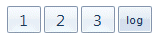Key sequence to find log10(123)

We know that the logarithm to base ten of one hundred is two, because two is the power to which we must raise ten in order to get one hundred (100 = 102). We also know that the logarithm to base ten of one thousand is three, because three is the power to which we must raise ten in order to get one thousand (1000 = 103).

It is therefore logical to expect the logarithm of one hundred and twenty-three to be somewhere between two and three, and since one hundred and twenty-three is considerably closer to one hundred than it is to one thousand, we could expect its common logarithm to be only marginally greater than two.

The answer we get confirms this theory, as you can see from the screenshot below. What we can also infer from this is that the logarithm to base ten of a real number with n digits preceding the decimal point will have a value that falls somewhere between n-1 and n.Log10(123)

## The laws of logarithms

Although we do not really use logarithmic tables any more, it would be a useful exercise to investigate just why they were so useful in the days before modern calculators were available. In order to do that we need to know about certain laws of logarithms (sometimes called logarithmic identities).

The laws of logarithms essentially help us to understand the relationship between logarithmic functions and power functions. You will recall, if you have looked at the page entitled "Powers and Roots", that there are a number of laws of indices. Hopefully you will be able to see as we progress that these laws are closely related to the laws of logarithms. Before we go any further, however, we should explain what we mean by logarithmic and power functions. A power function could take the form:

ƒ(x) = b x

The action of the function ƒ is to raise a base b to the power of x. The relationship between logarithmic functions and power functions is such that the logarithmic function is the inverse of the power function. What do we mean by that? The definition of the inverse of a function is that it reverses the action of the function. This means that if a function ƒ(x) produces an output b x, then the inverse function will produce an output x when applied to b x. To generalise, we can say that functions ƒ(x) and g(x) are inverses of one another if they satisfy the following conditions:

ƒ(g(x)) = x   and    g(ƒ(x)) = x

A logarithmic function takes the form:

ƒ(x) = logb (x)

So if the logarithmic function g(x) = logb (x) really is the inverse of the power function ƒ(x) = b x, we should be able to prove it with an example. Let's assume for the purposes of this exercise that we are using base ten, since this means we can use common logarithm notation (lg), and that our initial value of x is five.

10 lg(5) = 100.699 = 5   and   lg (105) = lg (100,000) = 5

It certainly seems, therefore, that functions ƒ(x) = b x and g(x) = logb (x) really are inverses of one another, but maybe you should try this with various values of x to satisfy yourself that it really is so. Getting back to the laws of logarithms, there are three important laws that, between them, answer the question we asked ourselves at the beginning of this section, which is why logarithms were so useful before the age of the electronic calculator.

## The first law of logarithms

The first law of logarithms states that the logarithm of the product of two numbers (i.e. the result of multiplying two numbers together) can be found by adding together their individual logarithms. This is expressed formally as:

logb (m × n) = logb (m) + logb (n)

The implication of this is that if we want to find the product of two numbers, we can do so without performing multiplication. Instead, we add together the individual logarithms of the two numbers, and take the antilogarithm of the result. The term antilogarithm does not refer to some sub-atomic particle thought to exist in another universe. It essentially means that, instead of looking up the logarithm for a particular number, we reverse the process and look up the number to which a particular logarithm refers.

An example will help to demonstrate how you can carry out such a calculation using the Microsoft Windows built-in calculator (or any suitable scientific calculator). Staying with base ten numbers and common logarithms for now, let's multiply five hundred and forty-seven by two hundred and thirty-nine (547 × 239). If you are using the calculator program built-in to Microsoft Windows, get the log to base ten of five hundred and forty-seven and store it in the calculator's memory by entering the following key sequence using the mouse:Take the common log of 547 and store it in memory

Your calculator window should now look like this: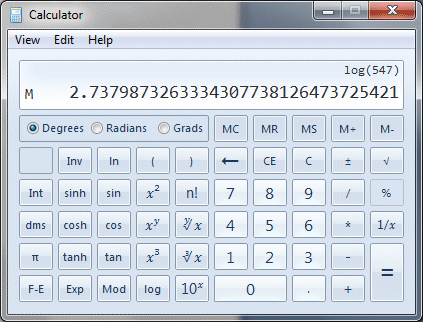The value of lg(547) has been stored in memory

Before continuing, press the Clear button (marked "C") to clear the display. Now get the log to base ten of two hundred and thirty-nine and store it in the calculator's memory by entering the following key sequence:Take the common log of 239 and add it to memory

Your calculator window should now look like this:The value of lg(239) has been added to memory

Press the Clear button once more to clear the display, then press the Memory Recall button (this is marked marked "MR"). Your calculator window should now look like this:Memory holds the sum of lg(547) and lg(239)

Don't clear the display just yet. We now have the sum of the two logarithms, but how do we take the antilogarithm of this value to get the product of five hundred and forty-seven and two hundred and thirty-nine? When you think about it, what we have is the log to base ten of the number we want, which means if we raise ten to the power of that number, we should get the correct answer.

As we have said before, a logarithm is simply an exponent (or power or index, whichever term you prefer). By definition, the logarithm to base ten of any number is the power to which we must raise ten to get that number. If you look on your Windows calculator you will see a button marked "10x" (in the version I have, this is in the middle of the bottom row of buttons).

This button raises ten to the power of whatever value is currently in the display. If you like, it is the antilog button for common logarithms (i.e. logarithms to base ten). If you are using your own scientific calculator, you might want to check which key or key sequence carries out the equivalent action. Make sure the value you retrieved from memory is still in the display, and click on the "10x" button. Your display should now look like this:The "antilog" button gives the required result

This is indeed the correct answer (547 × 239 = 130,733). Back in the 17th Century there were no calculators available, so logarithms (and antilogarithms) had to be looked up in tables. Even so, the process of multiplication could be reduced to a process of addition. Simply find the logarithms of the numbers you want to multiply, add them together, look up the antilogarithm and you have the answer.

The technique works equally well for multiplying more than two numbers. Simply add the logarithms of all of the numbers to be multiplied and find the antilogarithm. The main disadvantage of using log tables in this way was that the precision to which an answer could be obtained was limited by the precision of the log table values themselves, but the results were accurate enough for most purposes.

## The second law of logarithms

The second law of logarithms states that the logarithm of the quotient of two numbers (i.e. the result of dividing one number by another) can be found by subtracting the logarithm of the divisor (the number we are dividing by) from the logarithm of the dividend (the number we want to divide). This is expressed formally as:

logb (m ÷ n) = logb (m) - logb (n)

The implication of this is that if we want to find the quotient of two numbers, we can do so without performing division. Instead, we subtract the logarithm of the divisor from the logarithm of the dividend, and take the antilogarithm of the result. As an example, let's use the same numbers we used before but this time we will divide the number five hundred and forty-seven by the number two hundred and thirty-nine (547 ÷ 239).

If you are using the calculator program built-in to Microsoft Windows, get the log to base ten of five hundred and forty-seven and store it in the calculator's memory as you did before (make sure you clear the memory before you start). This time, we want to subtract the logarithm of two hundred and thirty-nine from the value stored in memory. Clear the display once more, and then enter the following key sequence: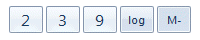Take the common log of 239 and subtract it from memory

Press the Clear button once more to clear the display, then press the Memory Recall button. Your calculator window should now look like this:The memory holds the difference of lg(547) and lg(239)

To obtain the answer, we need only take the antilogarithm of the value now held in the calculator's memory. Make sure the value you retrieved from memory is still in the display, and click on the "10x" button. Your display should now look like this:Click on the "antilog" button to get the result

This is indeed the correct answer (547 ÷ 239 = 2.289). As with multiplication using logarithms, we have simplified the process of division. This time the process has been reduced to one of subtraction. So, find the logarithms of the dividend and divisor, subtract the logarithm of the divisor from that of the dividend, look up the antilogarithm and you have the answer.

The technique works equally well for a number of division operations in a single expression, but you must be careful with the order of evaluation (division, unlike multiplication, is neither commutative nor associative). Consider the following expression:

336 ÷ 16 ÷ 4 = 5.25

The order of operations here is left to right, since only division operations are involved. The two divisors could be swapped around without affecting the result, but the dividend (336) must appear on the left-hand side of the expression, and the first division operation must always be evaluated before the second. Both of the expressions below give different results from the expression above. They also give different results from each other, as you can see.

336 ÷ (16 ÷ 4) = 84

336 ÷ (4 ÷ 16) = 1,344

This expression, on the other hand, gives us the same result as the original expression:

336 ÷ 4 ÷ 16 = 5.25

The original calculation can be carried out using logarithms like this:

Log10 336 - Log10 16 - Log10 4 = Log10 (336 ÷ 16 ÷ 4) = Log10 5.25

When I do this calculation using logarithms on the Windows calculator, I get the correct result, as shown below.The expected result (5.25) is achieved

## The third law of logarithms

The third law of logarithms states that the logarithm of a number raised to a power can be found by multiplying the logarithm of the base by the exponent (i.e. the power to which the base must be raised). This is expressed formally as:

logb (mn) = n logb (m)

The process of exponentiation (raising a base to a power) is reduced to one of multiplication. We multiply the logarithm of the base by the exponent, and take the antilogarithm of the result. As an example, let's use logarithms to base ten to find the value of one hundred and twenty-three to the power of five (1235). If you are using the calculator built-in to Windows, get the log to base ten of one hundred and twenty-three and multiply it by five using the following key sequence: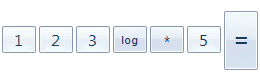Take the common log of 123 and multiply it by 5

Your calculator window should now look like this: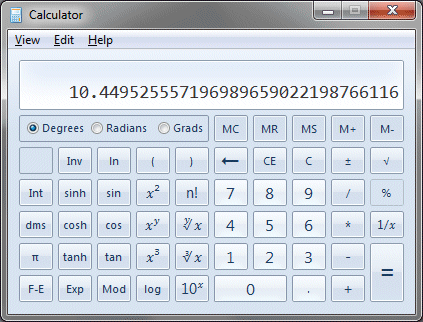The result of multiplying lg(123) by 5

To obtain the answer, we just take the antilogarithm of the value in the display by clicking on the "10x" button. Your display should now look like this:Use the "antilog" button to get the required result

This is indeed the correct answer (1235 = 28,153,056,843). The third law of logarithms can also be applied to finding roots, since a root is essentially a number raised to an exponent that is a rational (i.e. fractional) number. This relationship can be expressed as follows:

na  =  a 1/n

Translated into words, this means that the nth root of a is equal to a raised to the power of one over n. Remember, however, that the third law of logarithms states that the logarithm of a number raised to a power can be found by multiplying the logarithm of the base by the exponent. Therefore, to find the logarithm of the nth root of a number a, we simply take the logarithm of a and multiply it by  1/n. This can be expressed algebraically as:

 logb n√a  =  logb a1/n  =  logb a × 1 = logb a n n

As before, an example should serve to demonstrate the principle. Let's say that we want to find the cube root of nine hundred and seventy-five (3√975). Using a calculator, take the log of nine hundred and seventy-five and divide it by three. Now take the antilogarithm of the result. Using the Windows calculator, I get the result illustrated below:The antilogarithm gives us the cube root of 975

The Windows calculator has a button to find the value of x to the power of three (how convenient!), so clicking on this button now should get us back to nine hundred and seventy-five:Cubing the cube root gets us back to 975

We can extend the principle slightly to find the logarithm of rational powers in which the numerator m can be greater than one. Translating this into words, it means that a to the power m is raised to the power of one over n. Note that m can be any positive integer, including one. Since we already know that the logarithm of a number raised to a power can be found by multiplying the logarithm of the number by the exponent, we can modify the previous algebraic expression to make it slightly more generic as follows:

logb am/n  =  m logb a ÷ n

The third law of logarithms works equally well for numbers that are raised to a power that has a rational component. Consider the following example:

x = 8.754.76

This calculation is easy enough with a modern calculator, but would have been challenging in the days before calculators were available without the aid of logarithmic tables or a slide rule. We can perform the calculation using logarithms as follows:

log10 x = 4.76 × log10 8.75 = 4.483 . . .

We then take the antilogarithm of the result. This is what the calculation produces on the Windows calculator:Finding 8.75 to the power of 4.76 using logarithms

## Other logarithmic identities

In addition to the laws of logarithms outlined above, there are several other logarithmic identities and relationships that you should be aware of. These can be defined with respect to any base b such that:

logb (bn)  =  n

b1  =  b  ∴  logb b  =  logb b1  =  1

b0  =  1  ∴  logb 1  =  logb b0  =  0

logb a  is undefined if  a  =  0

logb a  is undefined if a is negative

## Changing the base of a logarithm

The laws of logarithms apply no matter what base we are using, and most of the calculations we have undertaken so far could have been done just as easily using natural logarithms (logarithms to base e) providing we had a calculator that could take the log to base e of a number and also get the antilog to base e.

Sometimes, however, we may be able to get the logarithm of a number in (say) base ten, but want to convert it to the logarithm to base two of that number (i.e. a binary logarithm). We can in fact convert a logarithm in a particular base n to a different base m, because the values are proportional. For any pair of bases m and n, it can be shown that:

 logm x  = logn x logn m

This means that if we wished to find the logarithm to base two of a particular value, we could use either common logarithms (log to base ten) or natural logarithms (log to base e) on the right-hand side of the equation. Let's say that we wanted to find the log to base two of one thousand. The calculation would be as follows:

 log2 1000  = log10 1000 log10 2

If you are using the calculator built-in to Windows, enter the following key sequence: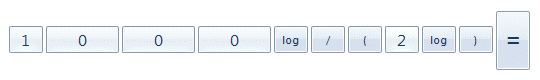Enter this key sequence to find log to base two of 1,000

Here is the output:The log to base two of 1,000

## Solving indicial equations

Solving equations is about finding the currently unknown value of one or more of the terms in the equation. Sometimes, the unknown value we are looking for in an equation is the index of an exponentiation (i.e. the power to which a number has been raised). Since logarithms are essentially exponents, we can use what we know about logarithms to solve such equations. Consider the following expression:

9.57 x = 78.36

Here, we are trying to find the power x to which nine-point-five-seven (9.57) must be raised in order to obtain the result seventy-eight-point-three-six (78.36). Since the values on both sides of the equation must be equal, we can start by taking the logarithm of both sides as follows:

log10 9.57 x = log10 78.36

Although we have used the logarithm to base ten here, the logarithm to any other base will work equally well. We know from the third law of logarithms that the logarithm of a number raised to a power can be found by multiplying the logarithm of the base by the exponent (i.e. the power to which the base must be raised), so we can re-write the above expression as:

x log10 9.57 = log10 78.36

We can now isolate x on the left-hand side of the equation, which means we can solve for x as follows (the answer here is given to three decimal places):

 x  = log10 78.36 =  1.931 log10 9.57

You can check the answer on your calculator by calculating the value of nine-point-five-seven to the power of one-point-nine-three-one (9.57 1.931).

## Natural logarithms

Natural logarithms are logarithms to base e. The letter e was introduced by 17th Century Swiss mathematician Leonard Euler to represent a mathematical constant, also known as Euler's number, which has an approximate value of 2.7182818284. The precise value of e cannot be calculated - it is an irrational number, meaning that its decimal expansion does not terminate and there is no repeating pattern.

The value of e is such that if we draw a graph of the function ƒ(x) = e x (known as the exponential function), the slope of the tangent line (known as the derivative) is equal to e x at any point on the graph, and is therefore equal to one when x is equal to zero. The illustration below shows the graph of function ƒ(x) = e x and the derivative at point x = 0. The constant e is often used in calculus because of its unique properties, and it occurs frequently in the natural world.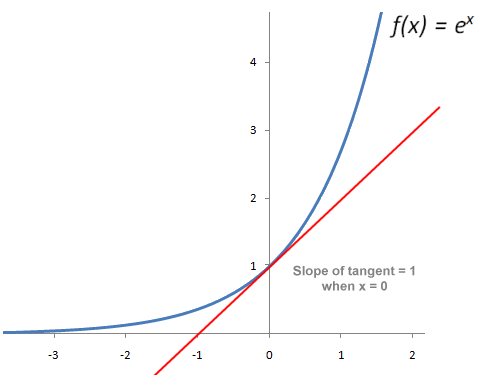A graph of the exponential function showing the derivative at x = 1

The exponential functions e x and e -x are of interest to engineers because they can be used to represent growth and decay (for example, the decay of an electrical charge stored in a capacitor through a constant load resistance).To get the natural logarithm of a number (i.e. the logarithm to base e) on a calculator, find the button marked "ln". For example, to find the natural logarithm of five hundred and seventy-eight (578) on the Windows calculator, use the following key sequence:Enter this key sequence to find the natural log of 578

Here is the output from the Windows calculator:The natural logarithm of 578

Finding the antilogarithm of a natural logarithm is not quite so straightforward on the Windows calculator, because the buttons you need for various inverse functions are hidden by default. Before we demonstrate how to do this, it is worth emphasising once again that the logarithmic function to any base is the inverse of the power function, and vice versa.

Thus, the inverse function of the logarithmic function ƒ(x) = loge x is the exponential function ƒ(x) = e x. Anyway, assuming you still have the natural logarithm of five hundred and seventy-eight in the calculator's display window, here is the key sequence to find the antilogarithm: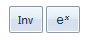Enter this key sequence to find an antilogarithm

Don't bother looking for the "ex" button before you start - it does not appear until after you have clicked on the "Inv" button, at which point it can be found immediately to the right of the "Inv" button. The "Inv" button toggles between various functions and their (inverse) counterparts, presumably because there is insufficient room in the application's window (which cannot be re-sized) to display all of the buttons at once. Here is the calculator's output after we have clicked on these keys:The antilogarithm of ln(578) produces 578

For the analysis of electrical circuits, e is often used in equations to find the transient value of a voltage or current in circuits where voltage and current change continuously over time. Consider the case where a capacitor with a capacitance C of twenty microfarads (20 × 10-6 Farads) is wired in series with a resistor with a resistance R of ten kilohms (10 × 103 Ohms) and a direct current (dc) power supply that supplies an initial supply voltage V of two hundred volts (200 volts).

For those who are unfamiliar with electrical principles, a capacitor is a circuit component that can hold a limited amount of electrical charge. The power supply provides the current that charges the capacitor, but the flow of current into the capacitor slows as the capacitor approaches its fully charged state, because the potential difference across the capacitor will increase and resist the flow of current.

If a resistor is in series between the power supply and the capacitor, the charging process takes longer, because the resistor reduces the rate at which current can flow in the circuit. For our purposes here, we are interested in the time t (in seconds) that must elapse between the moment at which we connect the resistor and (initially uncharged) capacitor with the power supply, and the point at which the transient voltage across the capacitor reaches one hundred volts.

All you really need to understand here is the equation used to determine the transient voltage v at any given point in time, which makes use of the exponential function and can be stated as:

v = V( 1 - e -t/CR )

We can substitute the known values in the equation to give us:

100 = 200 × ( 1 - e -t/0.0002 × 10,000 ) = 200 × ( 1 - e-t/0.2 )

We can re-arrange and simplify this equation as follows:

100 ÷ 200 = 1 - e -t/0.2

0.5 = 1 - e -5t

e -5t = 1 - 0.5 = 0.5

We can now put the equation in logarithmic form:

loge 0.5 = -5t

We can now rearrange the equation once more to get t onto the left-hand side of the equation, which will also give us an expression that we can easily enter into a calculator:

 t = - loge 0.5 5

Here is the key sequence on the Windows calculator:The key sequence for log to base e of 0.5 all over 5, negated

And here is the resulting calculator display:The elapsed time is 0.139 seconds to three significant figures

From the above, then, we can determine that for the circuit described, the transient potential difference across the capacitor will have reached a value of one hundred volts after an elapsed time (to three significant figures) of zero-point-one-three-nine seconds (0.139 seconds) after the circuit is connected with the power supply.

This kind of calculation is frequently required for the analysis of circuits involving resistors and capacitors in series, as well as for other kinds of circuits found in electrical and electronic systems. Since such circuits are used in pretty much every kind of electronic device you can think of, the ability to manipulate equations using natural logarithms is invaluable.

## Applications of logarithms

We have already seen how the rate of growth or decay of a current or voltage in an electrical circuit can be expressed using the exponential function. The use of logarithmic scales to express values that tend to grow or decay exponentially is found in any number of applications, some of which will be familiar to you. Earlier we mentioned the Richter Scale, which is used to express the magnitude of the seismic waves produced by earthquakes.

Another familiar unit of measurement based on logarithmic scales is the decibel (dB). Virtually everybody who has not spent their entire life in a cave with very little contact with the outside world has at least heard of the decibel (no pun intended). Most people will associate decibels with the level of sound they experience, although they may not fully understand the implications of an increase in sound level of single decibel.

The fact is that with regard to sound, human beings perceive an increase in sound levels logarithmically. This means that when you or I perceive that a sound (i.e. the neighbours next door having a row, or the volume produced by a loudspeaker) has become twice as loud, the magnitude of the sound pressure (i.e. the pressure wave created by the source of the sound) may have increased by a much greater factor.

The sound pressure level scale defines sound level in terms of the average variation in pressure in a medium such as air. It defines the amplitude of a sound as a measure of the average variation in pressure, measured in Pascals (Pa). The sounds we encounter on a daily basis are inherently complex, often comprising a very wide range of frequencies.

The quality of a sound is also highly subjective from a human point of view. Nevertheless, we can express the loudness of a sound, in terms of its likely effect on the human auditory system, in terms of either Pascals or decibels. The smallest amplitude that creates any sensation at all is about plus or minus twenty micro Pascals (± 20 µPa). This level represents the threshold of hearing (i.e. the smallest sound which we can hear under ideal conditions) and is used as the zero reference level in the sound pressure level scale (i.e. 0 dB).

A logarithmic scale is used because the human ear is far more sensitive to variations in sound at the lower end of the scale. Indeed, at a level of around plus or minus two hundred Pascals (± 200 Pa) sound can cause physical damage to the human auditory system (this is sometimes called the hearing pain threshold). Since each twenty decibel interval on the sound pressure level scale represents a tenfold increase pressure variation, this puts the hearing pain threshold at around one hundred and forty decibels (140 dB = ± 200 Pa).

To put this into some kind of perspective, the sound of a shotgun can register at between one hundred and fifty and one hundred and sixty decibels (150-160 dB), human eardrums can rupture at between one hundred and ninety and one hundred and ninety-five decibels (190-195 dB), and the sonic boom produced by the space shuttle at a speed of mach twenty during its landing approach has been measured at some two hundred and twenty decibels (220 dB).

Some of the loudest sounds ever recorded (even louder than nuclear detonations, in fact) have been created by violent volcanic eruptions. The eruption of Krakatoa in 1883 is estimated to have produced a sound level of around three hundred and ten decibels (310 dB = 63,245,553,203.36 Pa).

We can clearly see from the above examples that using a logarithmic scale often gives us a more meaningful way of representing values that can be very small at one end of a scale and almost unimaginably large at the other end. In the same way that logarithms reduce the complexity of an operation by one level (i.e. multiplication becomes addition and exponentiation becomes multiplication), using logarithms also allows us to turn an exponential scale of values into a more linear one.

Further examples of the use of logarithmic scales include their use in telecommunications to represent the loss of signal strength in transmission systems, and in astronomy to represent the relative luminosity of stars and other celestial objects. In fact, in any field in which values can be seen to vary along an exponential scale, we are likely to find logarithmic scales being used to represent them.